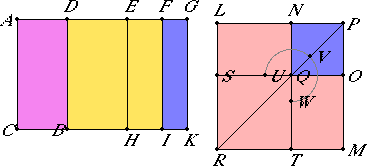# Proposition 96

If an area is contained by a rational straight line and a sixth apotome, then the side of the area is a straight line which produces with a medial area a medial whole.

Let the area AB be contained by the rational straight line AC and the sixth apotome AD.

I say that the side of the area AB is a straight line which produces with a medial area a medial whole.X.Def.III.6

Let DG be the annex to AD. Then AG and GD are rational straight lines commensurable in square only, neither of them is commensurable in length with the rational straight line AC set out, and the square on the whole AG is greater than the square on the annex DG by the square on a straight line incommensurable in length with AG.

X.18

Since the square on AG is greater than the square on GD by the square on a straight line incommensurable in length with AG, therefore, if there is applied to AG a parallelogram equal to the fourth part of the square on DG and deficient by a square figure, then it divides it into incommensurable parts.

Bisect DG at E, apply to AG a parallelogram equal to the square on EG and deficient by a square figure, and let it be the rectangle AF by FG. Then AF is incommensurable in length with FG.

X.11

But AF is to FG as AI is to FK, therefore AI is incommensurable with FK.

X.21

Since AG and AC are rational straight lines commensurable in square only, therefore AK is medial. Again, since AC and DG are rational straight lines and incommensurable in length, DK is also medial.

Now, since AG and GD are commensurable in square only, therefore AG is incommensurable in length with GD.

But AG is to GD as AK is to KD, therefore AK is incommensurable with KD.

VI.26

Now construct the square LM equal to AI, and subtract NO, equal to FK, about the same angle. Then the squares LM and NO are about the same diameter.

Let PR be their diameter, and draw the figure. Then in manner similar to the above we can prove that LN is the side of the area AB.

I say that LN is a straight line which produces with a medial area a medial whole.

Since AK was proved medial and equals the sum of the squares on LP and PN, therefore the sum of the squares on LP and PN is medial. Again, since DK was proved medial and equals twice the rectangle LP by PN, therefore twice the rectangle LP by PN is also medial.

Since AK was proved incommensurable with DK, therefore the sum of the squares on LP and PN is also incommensurable with twice the rectangle LP by PN. And, since AI is incommensurable with FK, therefore the square on LP is also incommensurable with the square on PN.

Therefore LP and PN are straight lines incommensurable in square which make the sum of the squares on them medial, twice the rectangle contained by them medial, and further, the sum of the squares on them incommensurable with twice the rectangle contained by them.

X.78

Therefore LN is the irrational straight line called that which produces with a medial area a medial whole, and it is the side of the area AB. Therefore the side of the area is a straight line which produces with a medial area a medial whole.

Therefore, if an area is contained by a rational straight line and a sixth apotome, then the side of the area is a straight line which produces with a medial area a medial whole.

Q.E.D.

## Guide

This proposition is used in X.110.